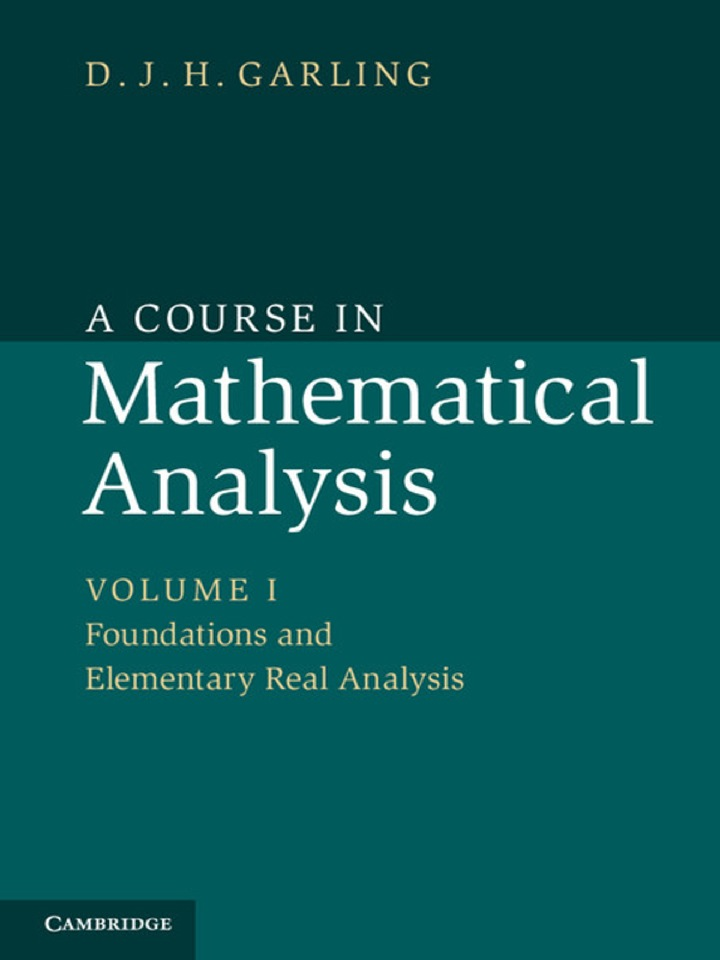# A Course in Mathematical Analysis: Volume 1, Foundations and Elementary Real Analysis

## A Course in Mathematical Analysis: Volume 1, Foundations and Elementary Real Analysis

By D. J. H. Garling

Expires on Nov 26th, 2021
\$41

Publisher List Price: \$0.00

The three volumes of A Course in Mathematical Analysis provide a full and detailed account of all those elements of real and complex analysis that an undergraduate mathematics student can expect to encounter in their first two or three years of study. Containing hundreds of exercises, examples and applications, these books will become an invaluable resource for both students and instructors. This first volume focuses on the analysis of real-valued functions of a real variable. Besides developing the basic theory it describes many applications, including a chapter on Fourier series. It also includes a Prologue in which the author introduces the axioms of set theory and uses them to construct the real number system. Volume 2 goes on to consider metric and topological spaces and functions of several variables. Volume 3 covers complex analysis and the theory of measure and integration.

Subject: Mathematics & Statistics -> Mathematics -> Mathematics General1st edition
Publisher: Cambridge University Press 4/25/13
Imprint: Cambridge University Press
Language: English

ISBN 10: 1107301858
ISBN 13: 9781107301856
Print ISBN: 9781107032026

Live Chats